Archived

This topic is now archived and is closed to further replies.

Recommended Posts

Hello, Survivors!

Each week we run community contests for all players (all platforms included where possible) where you can win lots of cash prizes! Below is the different type of contests that take place. Try your hand and share some of your creations with us today!

ARK NVIDIA Ansel Photography Contest!

This weekly screenshot competition asks players to take in-game photographs from either ARK: Survival Evolved or our new desert themed expansion pack ARK: Scorched Earth using NVIDIA Ansel, and meet the category requirements listed below.

Winners will be announced during ARK’s regular “Community Crunch” blog post. Each winner (3) will receive \$100 each and each runner up will receive \$50. Want to enter? ARK-Ansel screenshot entries should be e-mailed to anselcontest@studiowildcard.com

If you need a place to upload a file for the contest, make use of Wetransfer which will allow up to 2gb for free!

Please note: Ansel submissions are limited to a total of 15 per person!

Three Categories

• Super Resolution: These should be taken at 8x Super Resolution. Players can optionally use Depth of Field effects accessible in Spectator mode by pressing the [Delete] key.
• Panoramic 360 Stereoscopic 3D: These should be taken at 4x Super Resolution,  in 360 Stereoscopic 3D mode. We will crop them to 2D for web display, while also providing the 3D version for viewing within VR display. Check out your 360 Stereo results on your HMD or on your Android Phone with NVIDIA VR Viewer
• Freeform: Any settings, whacky and creative use of Ansel filters are encouraged. Adding humorous titles/captions in Photoshop is also acceptable for this category!

When entering the contest please name your entries and make sure that they include:

• Name
• Image Title
• Category

An example would be

Wildcard Jat - Evolution Event - Super Resolution

Ansel is a revolutionary new way to capture in-game shots.  Compose a shot from any position, adjust with post-process filters, capture HDR images in high-fidelity formats, and share the screenshots in 360 via a smartphone, PC or VR headset. Learn more about these features in the Ansel Technology page and the NVIDIA blog.

How to run NVIDIA Ansel

First of all, you'll want to make sure you're running the latest Nvidia Geforce Drivers - so head over to http://www.geforce.com/ and get downloading!

Once setup and ready to go, right click ARK: Survival Evolved in your steam library and you'll see the option to Launch ARK with NVIDIA Ansel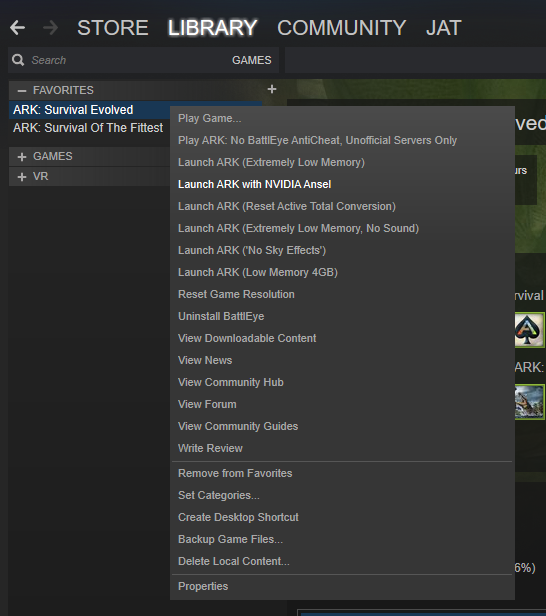Select this and head into a single player game, regardless of the map or the mod. Once you're in game, you can immediately press ALT + F2 to get into Ansel Photography mode and start taking snaps.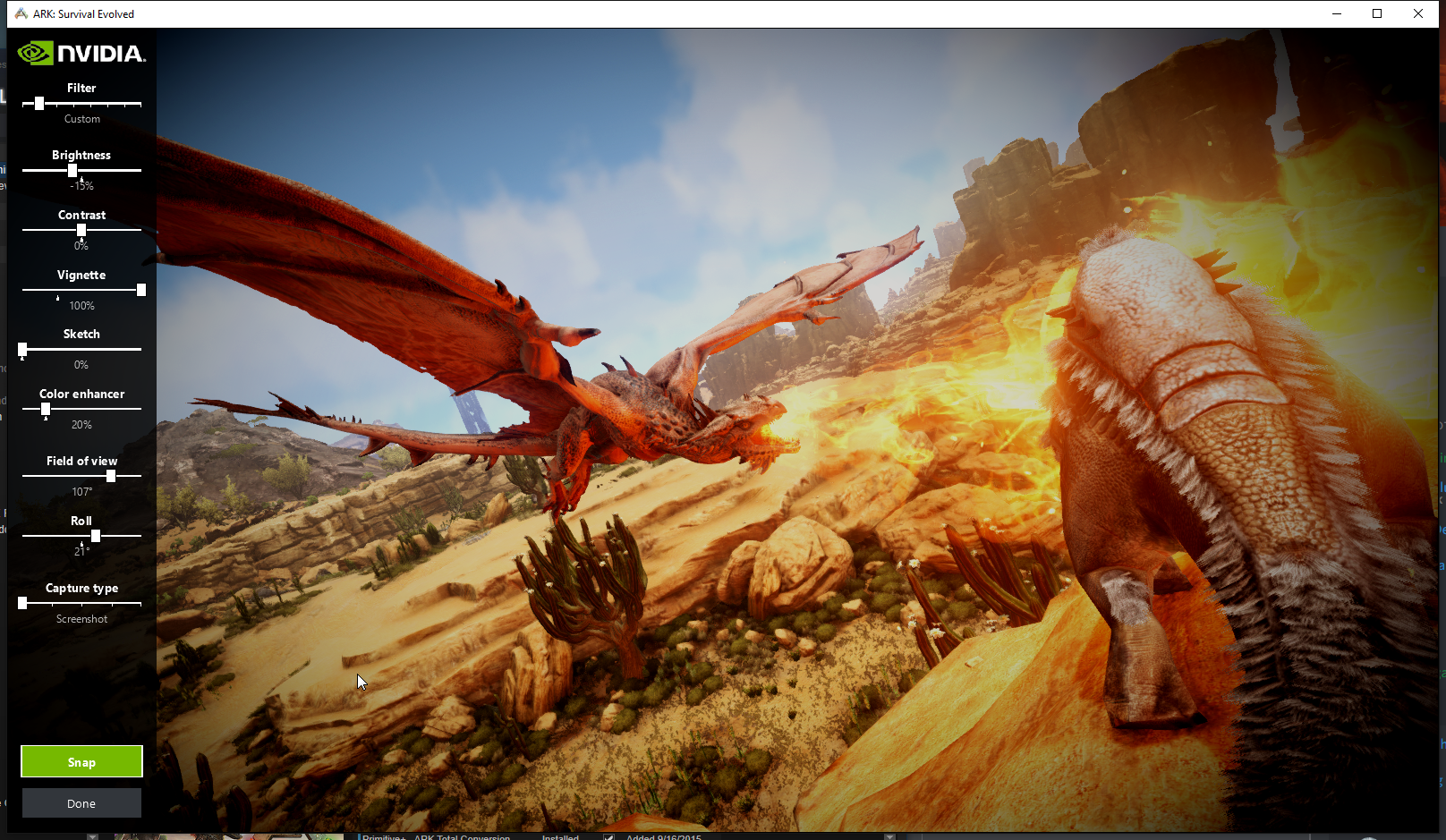or you can type in the following command in the console:

cheat enablespectator

Once that is enabled, hit your DELETE key and it'll open the Depth of Field Options menu where you can play around with the games DOF.Then hit ALT+F2 and take some awesome Ansel photography!

Here's a little tip from Jesse:

Quote

You can also type "enablespectator true" in the console to fly around before you take your shot, and hit the "Home" key to level your camera if you want to keep people from feeling sick on a 360 stereo VR screenshot.

Procedurally Generated Maps!Welcome to the newest contest in our Community Crunch, PGMs! Players will be able to show off their creations for a chance to win a 100\$ Steam Gift card or \$50 cash for runners-up, plus get their map featured in the next set of Official Extinction server maps! That's right, your map could be featured as an Official Server for a one month period!

Please include the following in your entry post:

• Map Name:
• Media (Videos, Screenshots, etc):
• PGM Settings:

Media content of your procedurally generated map can include videos, screenshots, and live stream vods - anything you want in which we'll be able to visually see how your map looks. You can even get players on it and have them build or battle out a PvP game to show what it's capable of!

The PGM settings used to create the map can be found your game.ini - That's most likely in:

Steam\steamapps\common\ARK\ShooterGame\Saved\Config\WindowsNoEditor\Game.ini

or

\Saved\Config\Windows or WindowsServer or LinuxServer etc)\Game.ini

[/Script/ShooterGame.ShooterGameMode]
PGMapName= ...
PGTerrainPropertiesString= ...

For extra details about PGM, check out this thread here:

And of course, if you are using a special modded Procedural ARK Generator, let us know and share it with us!

ARKitect!

This is an award (\$200 prize for first and \$100 for any runners up) given to Survivors to celebrate the amazing structures you build. These bases are judge based upon a number of different factors such as their creativity, uniqueness, how fun of a base it is, the difficulty of building it, the functionality it provides and much more! At the end of each week, the winners and runner-ups will be featured in our #ARKitect section of the Community Crunch and will be awarded cash prizes!

Note: We've had a lot of requests regarding the use of mods - go for it! We'd love to see how mods have influenced your bases

ARKVideo!

This contest is open to all types of video be they funny, epic, scenic, stories, whatever inspires you. Winning this content will net you a \$200 prize for first and \$100 for any runners up. Just a general reminder that they should remain safe for work, think of the children! Send us in your videos and we cannot wait to watch them! We're really interested in seeing the latest video creations you guys have put together - so show us something fresh!

Please upload the images to the gallery section of our New Community Hub - you can create your own album and directly link us to that or attach it to your post! Please remember to post your entry to this thread specifically! If you're not able to upload directly to the gallery, don't sweat as imgur will work fine too!

Looking forward to seeing the entries!

Share on other sites

Carrying on my servers Boss mission!

Name: GP
Title: Beta Broodmother

This is an unofficial server of the Beta Broodmother fight post-balance changes for the Broodmother. This includes the new changes to the arena, i.e. no bridge. We also included a Yutyrannus for it's special ability.

Share on other sites

Using OBS as my video recorder now, the old one refused to work anymore. Still figuring this one out but it looks like it got the job done, except it plays the audio I am listening toARKitect Submission:

********Single, Combined Entry (Stages 1,2,3)*******

Classic Donkey Kong Arcade (Stages 1-3)

This is ONE ENTRY, combined. I didn't know how to combine videos captures using this software.

I made some small adjustments to fit ARK and make it playable. A fun, retro themed creation, this seemed so hard on DK64 back in the day.

Images: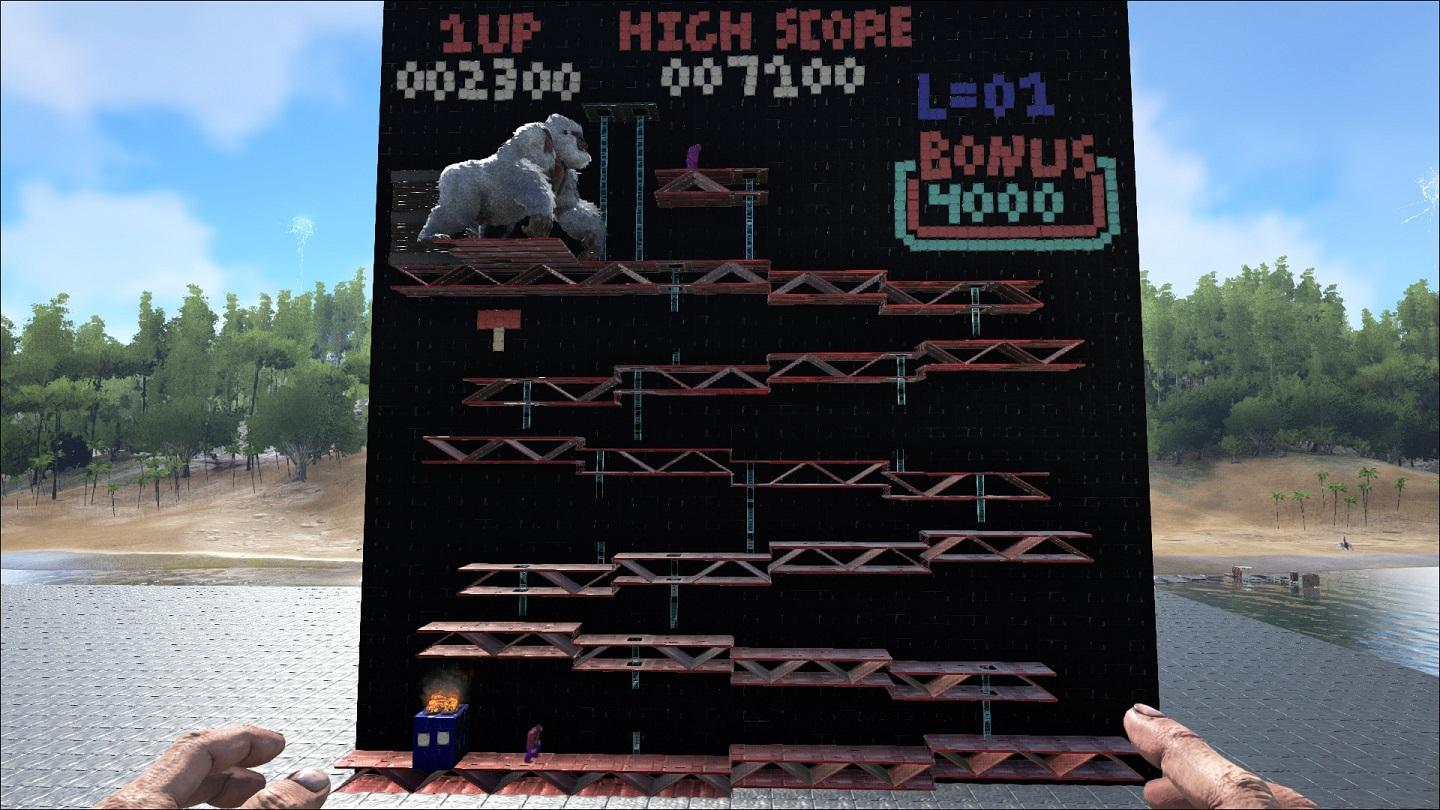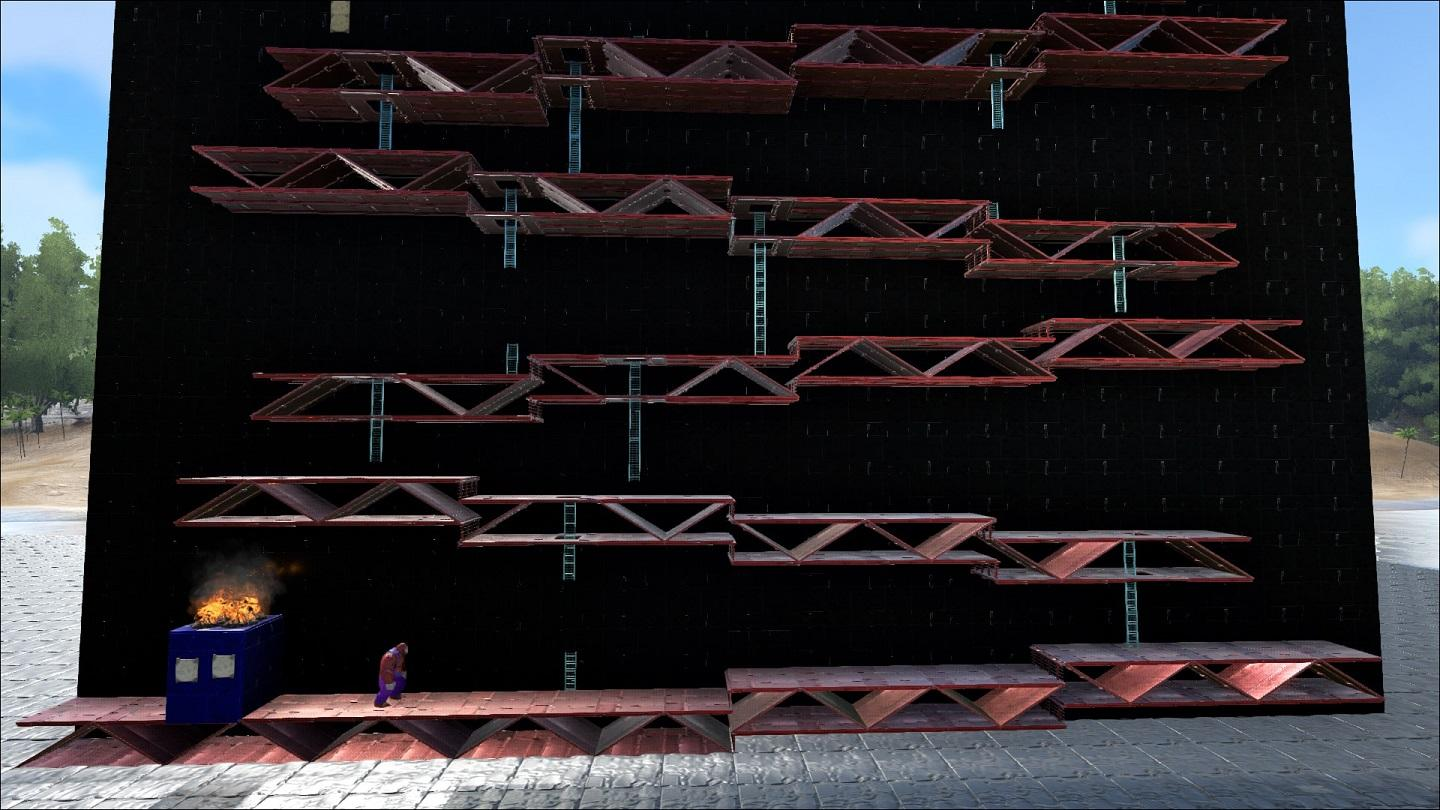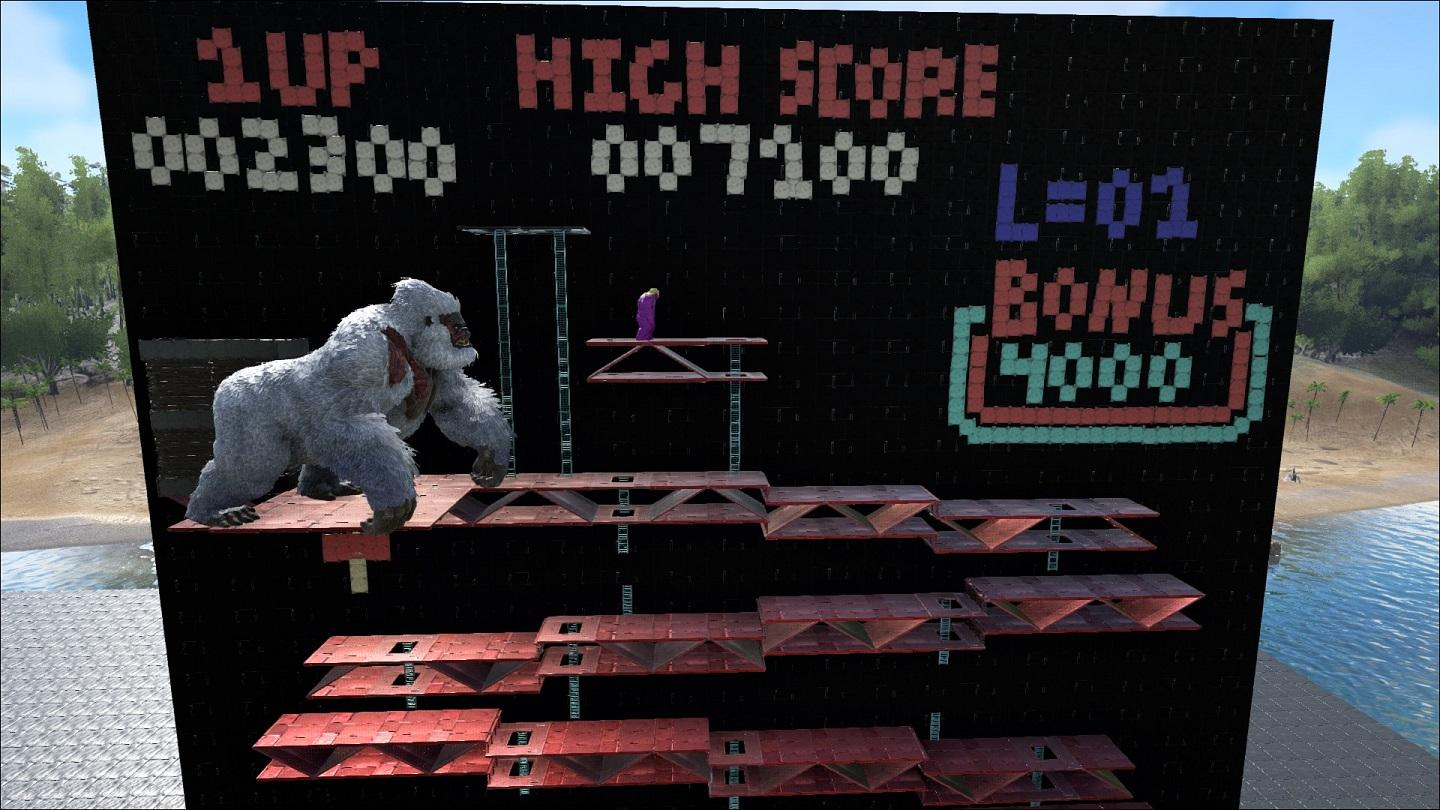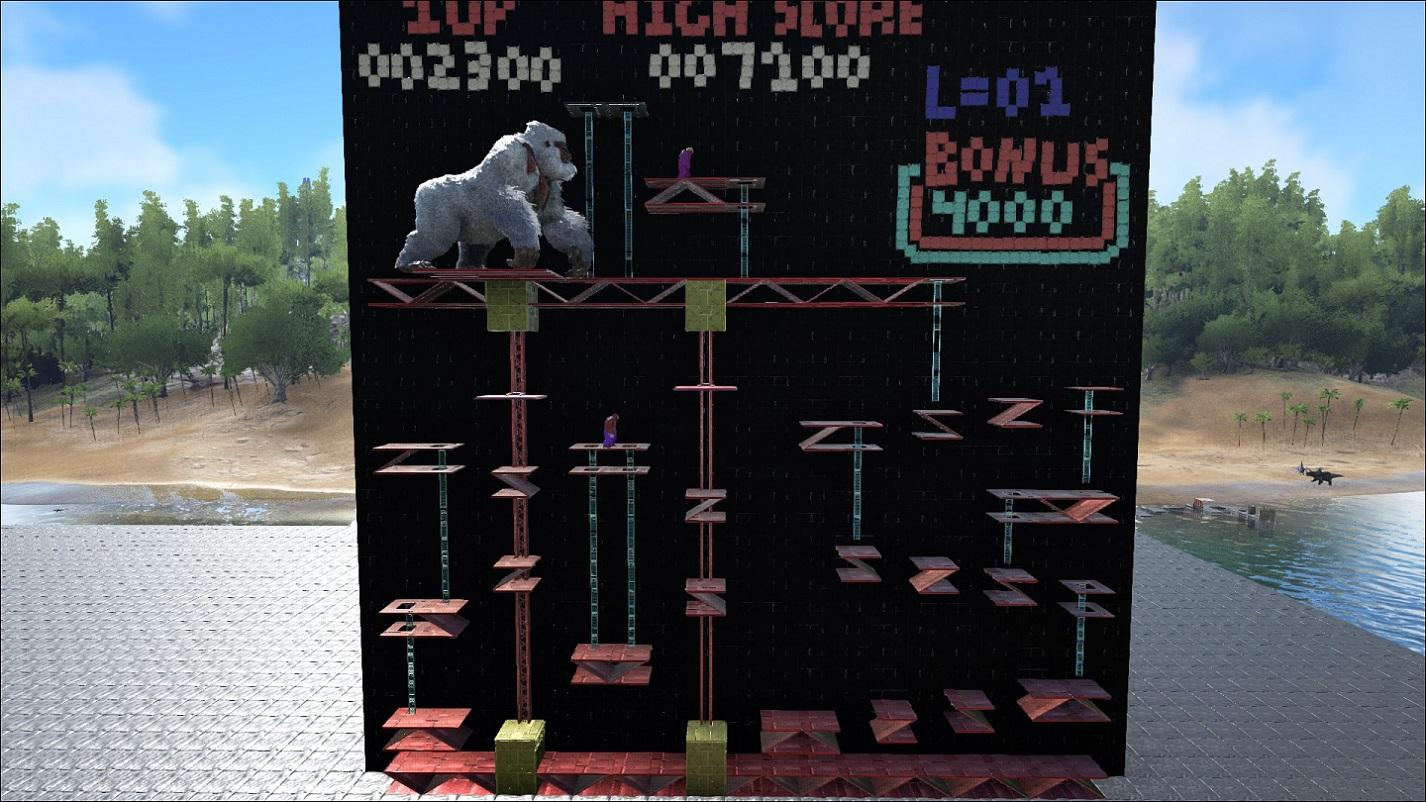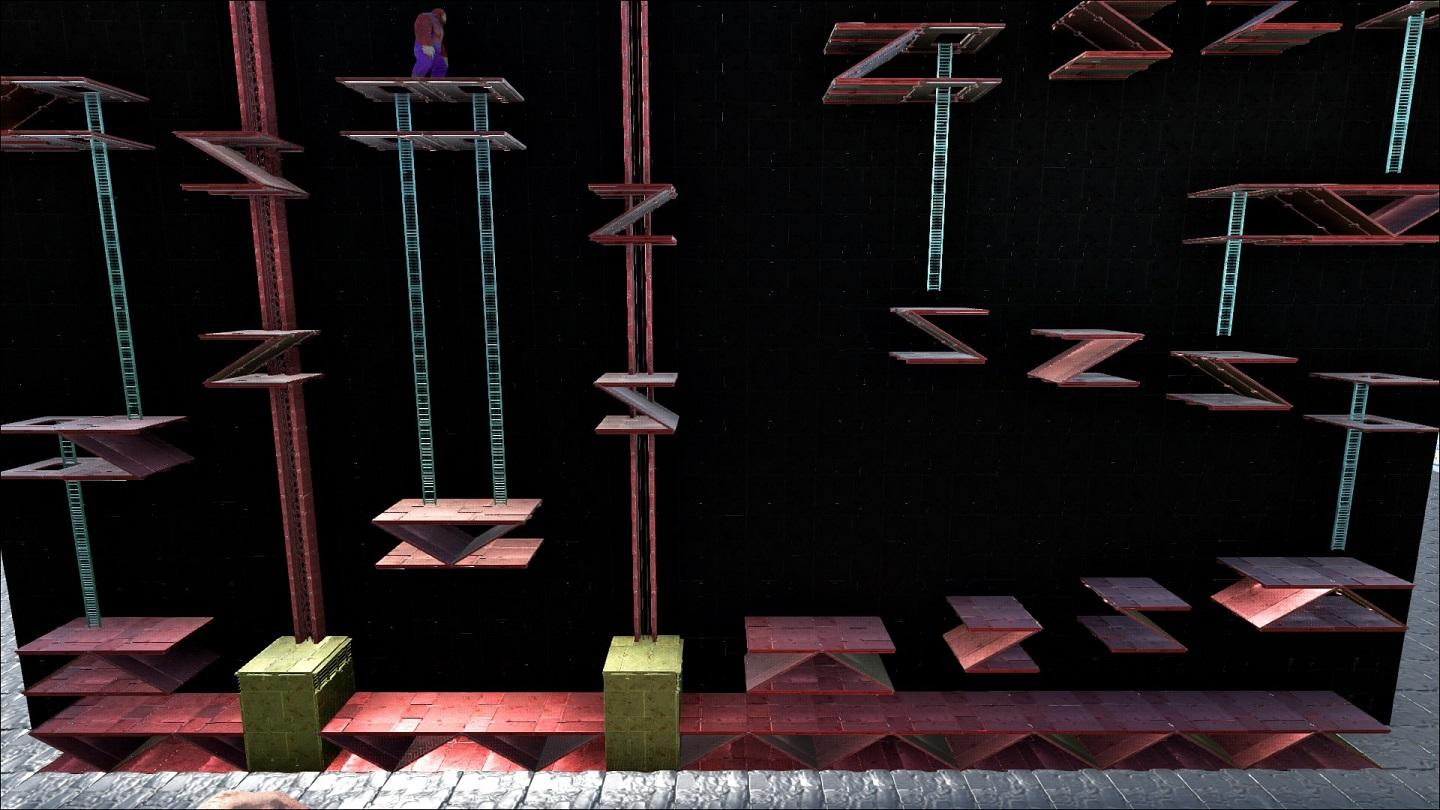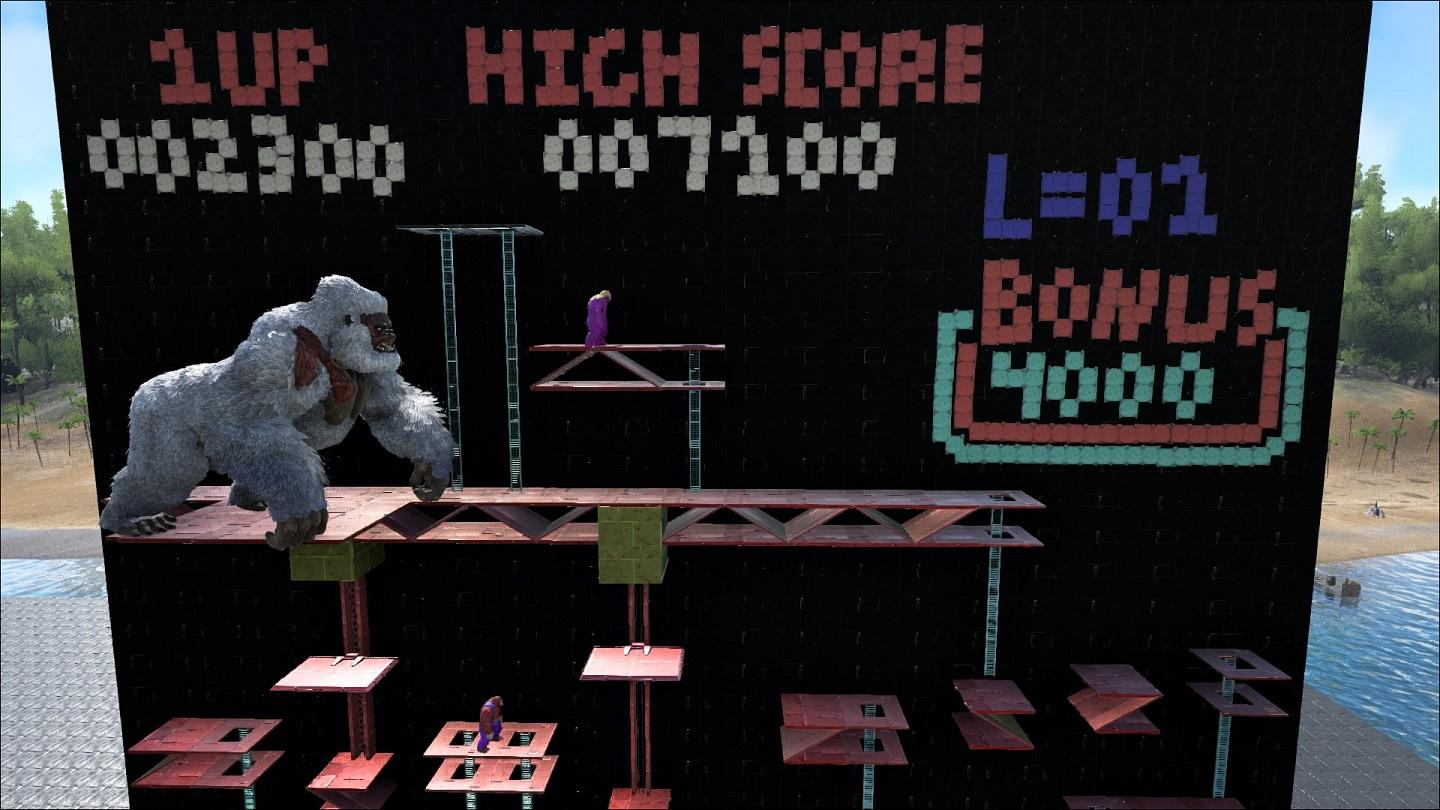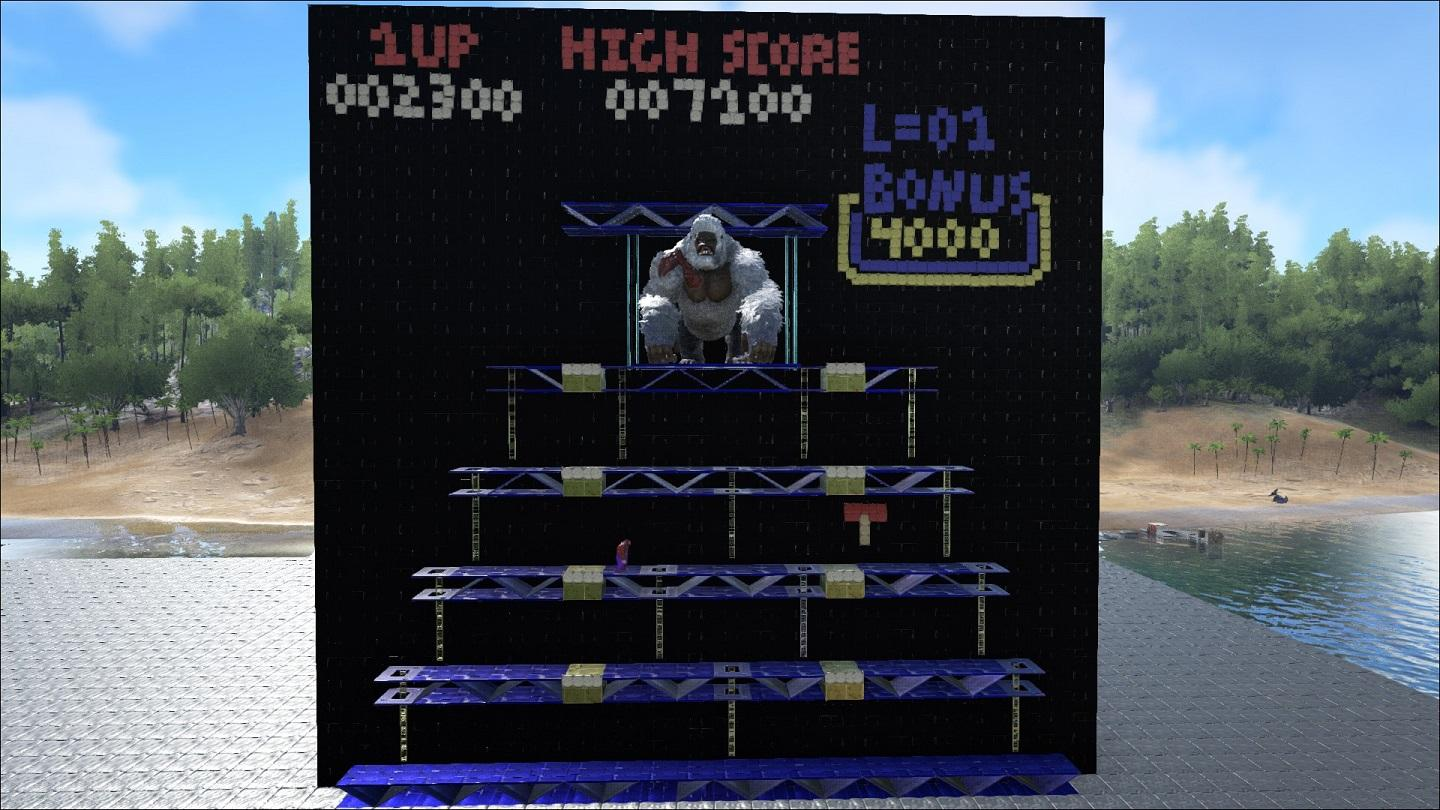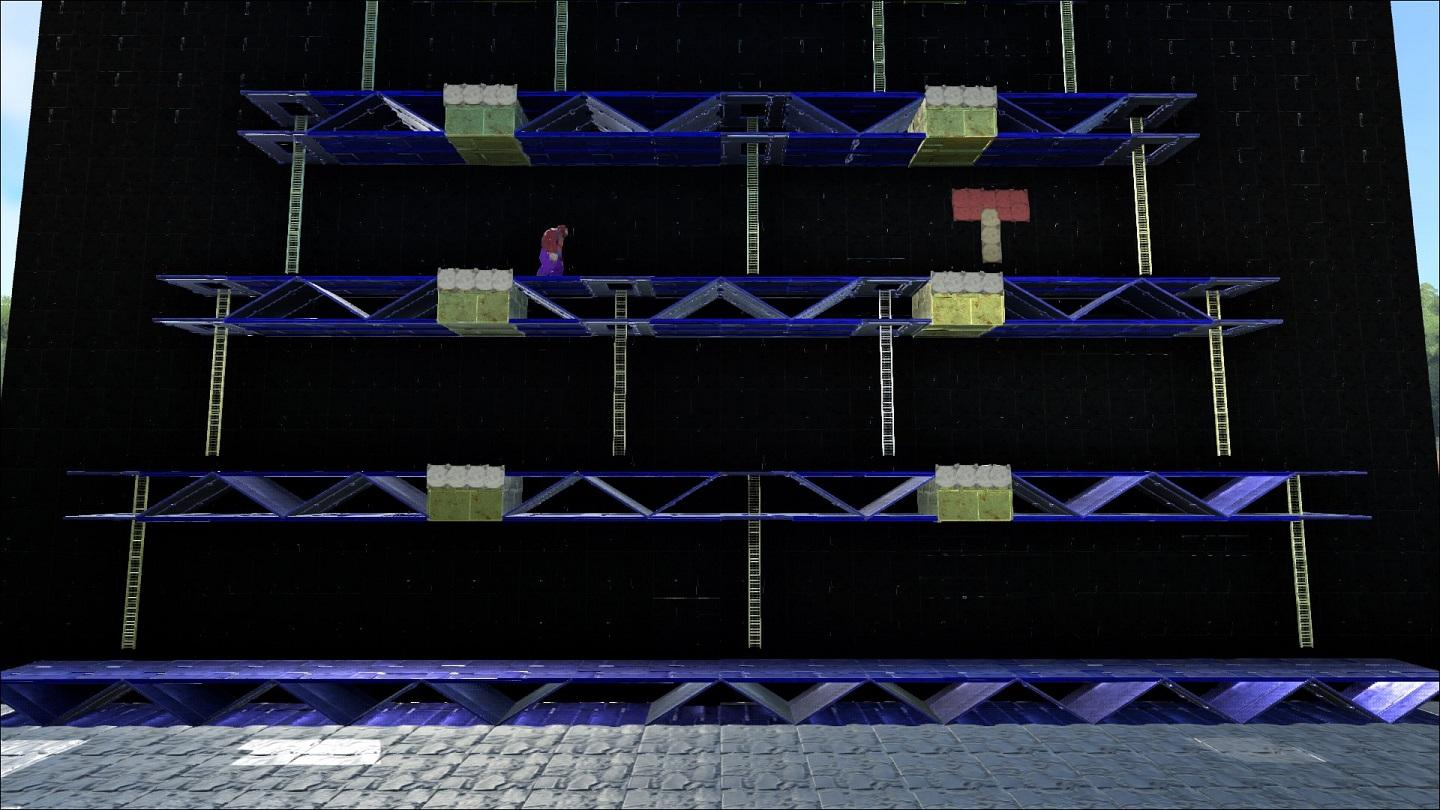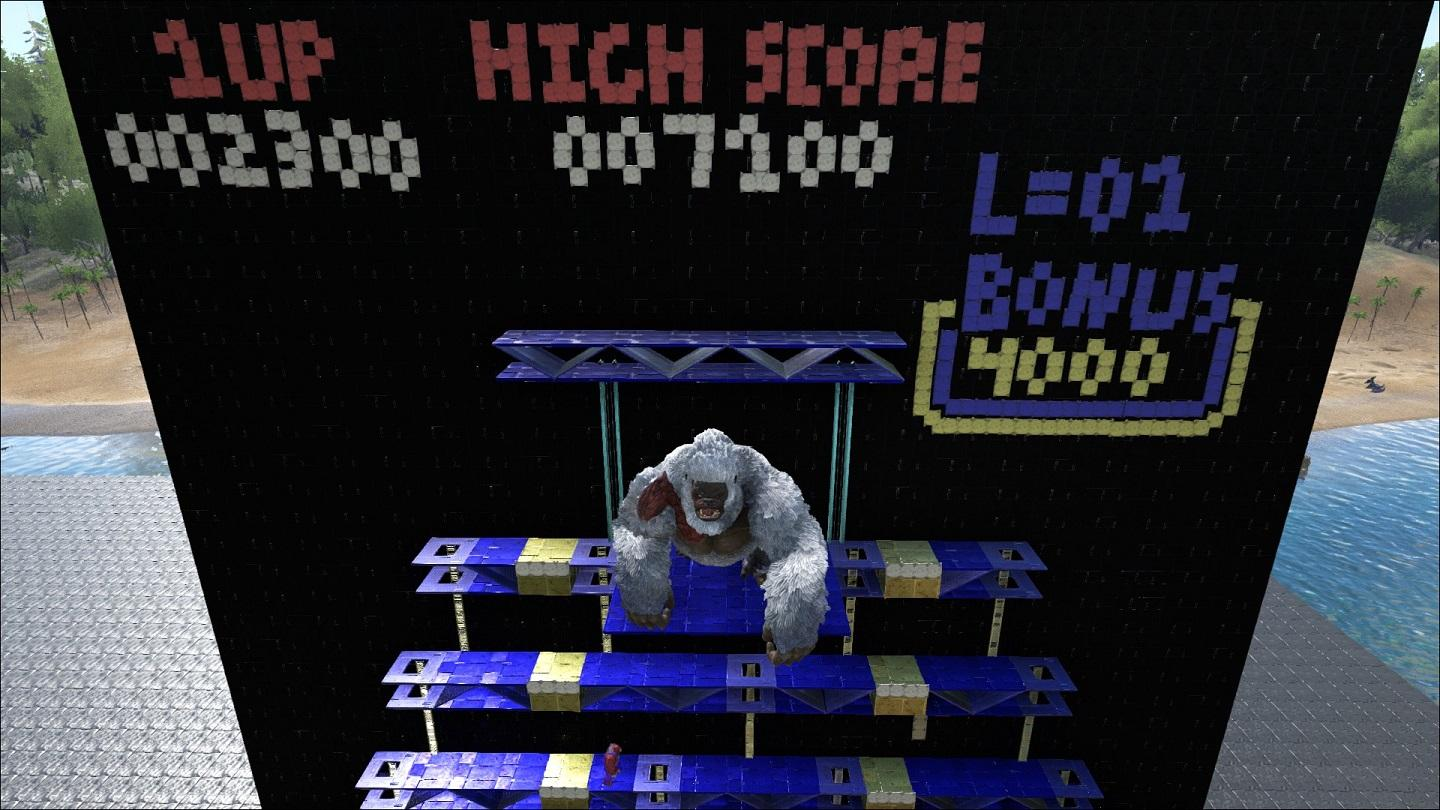Reference Images (in case someone isn't familiar with these)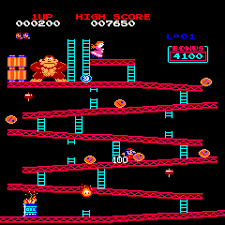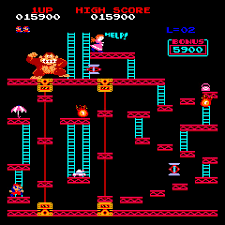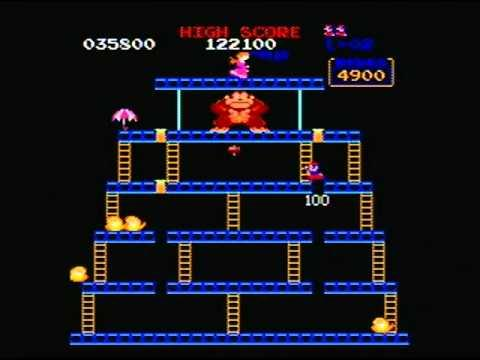Video Tours (Short)

Stage 1

Stage 2

Stage 3

PGM Submission:

Settings:
PGTerrainPropertiesString=MapSeed=907;LandscapeRadius=1.0;Water Frequency=3.1;Mountains Frequency=12;Mountains Slope=1.95;MountainsHeight=.78;Turbulence Power=0.0125;Shore Slope=1.4;WaterLevel=-0.72;GrassDensity=1.0;JungleGrassDensity=0.05;ErosionSteps=10;TreesGroundSlopeAccuracy=0.5;DepositionStrength=0.05;ErosionStrength=0.2;OceanFloorLevel=-1.0;SnowBiomeSize=0.14;RWBiomeSize=0.09;MountainBiomeStart=-0.49;MountainsTreeDensity=0.06;JungleBiomeStart=-0.59;IslandBorderCurveExp=4.0;MaxSawnPointHeight=0.2;SnowGrassDensity=0.25;MountainGrassDensity=0.007;SnowMountainGrassDensity=0.15;UnderwaterObjectsDensity=0.5;SnowMountainsTreeDensity=0.03;TreeDensity=0.005;JungleTreeDensity=0.50;RedWoodTreeDensity=0.010;SnowTreeDensity=1.2;RedwoodGrassDensity=0.07;ShoreTreeDensity=0.32;SnowShoreTreeDensity=0.04;DeepWaterBiomesDepth=-0.24;InlandWaterObjectsDensity=0.5;ShorelineStartOffset=0.023;ShorelineThickness=0.035;MountainGeneralTreesPercent=0.15;TerrainScaleMultiplier=(X=1.0f,Y=1.0f,Z=1.0f);SnowBiomeLocation=(X=0.6f,Y=0.6f);RWForestBiomeLocation=(X=0.35f,Y=0.35f);NorthRegion1Start=(X=0.25f,Y=0.0f);NorthRegion1End=(X=0.416f,Y=0.5f);NorthRegion2Start=(X=0.416f,Y=0.0f);NorthRegion2End=(X=0.582f,Y=0.5f);NorthRegion3Start=(X=0.582f,Y=0.0f);NorthRegion3End=(X=0.75f,Y=0.0f);SouthRegion1Start=(X=0.25f,Y=0.5f);SouthRegion1End=(X=0.416f,Y=1.0f);SouthRegion2Start=(X=0.416f,Y=0.5f);SouthRegion2End=(X=0.582f,Y=1.0f);SouthRegion3Start=(X=0.582f,Y=0.5f);SouthRegion3End=(X=0.75f,Y=1.0f);EastRegion1Start=(X=0.75f,Y=0.0f);EastRegion1End=(X=1.0f,Y=0.333f);EastRegion2Start=(X=0.75f,Y=0.333f);EastRegion2End=(X=1.0f,Y=0.666f);EastRegion3Start=(X=0.75f,Y=0.666f);EastRegion3End=(X=1.0f,Y=1.0f);WestRegion1Start=(X=0.0f,Y=0.0f);WestRegion1End=(X=0.25f,Y=0.333f);WestRegion2Start=(X=0.0f,Y=0.333f);WestRegion2End=(X=0.25f,Y=0.666f);WestRegion3Start=(X=0.0f,Y=0.666f);WestRegion3End=(X=0.25f,Y=1.0f)

Share on other sites

eco has put out a couple of additions to her popular Terrariums mod since I last did a showcase for it, so I took a few minutes to put together an update video.

ECO'S TERRARIUMS UPDATE

Share on other sites

This is my PGM for this week. I used the time code from Futurama as the seed lolNot sure why it seems to have changed in the ini settings though..[/Script/ShooterGame.ShooterGameMode]
PGMapName=ProcdeuralARK20170614121057
PGTerrainPropertiesString=MapSeed=-2147483648;LandscapeRadius=1.0;Water Frequency=5.0;Mountains Frequency=12.0;Mountains Slope=1.8;MountainsHeight=1.25;Turbulence Power=0.012;Shore Slope=1.0;WaterLevel=-0.72;GrassDensity=1.0;JungleGrassDensity=0.037;ErosionSteps=4;TreesGroundSlopeAccuracy=0.5;DepositionStrength=0.5;ErosionStrength=0.75;OceanFloorLevel=-1.0;SnowBiomeSize=0.26;RWBiomeSize=0.17;MountainBiomeStart=-0.55;MountainsTreeDensity=0.014;JungleBiomeStart=-0.65;IslandBorderCurveExp=4.0;MaxSawnPointHeight=0.1;SnowGrassDensity=0.18;MountainGrassDensity=0.062;SnowMountainGrassDensity=0.24;UnderwaterObjectsDensity=0.65;SnowMountainsTreeDensity=0.018;TreeDensity=0.0043;JungleTreeDensity=0.57;RedWoodTreeDensity=0.18;SnowTreeDensity=1.0;RedwoodGrassDensity=0.18;ShoreTreeDensity=0.046;SnowShoreTreeDensity=0.018;DeepWaterBiomesDepth=-0.24;InlandWaterObjectsDensity=0.45;ShorelineStartOffset=0.01;ShorelineThickness=0.0015;MountainGeneralTreesPercent=0.1;TerrainScaleMultiplier=(X=1.0f,Y=1.0f,Z=1.0f);SnowBiomeLocation=(X=0.2f,Y=0.2f);RWForestBiomeLocation=(X=0.5f,Y=0.5f);NorthRegion1Start=(X=0.25f,Y=0.0f);NorthRegion1End=(X=0.416f,Y=0.5f);NorthRegion2Start=(X=0.416f,Y=0.0f);NorthRegion2End=(X=0.582f,Y=0.5f);NorthRegion3Start=(X=0.582f,Y=0.0f);NorthRegion3End=(X=0.75f,Y=0.0f);SouthRegion1Start=(X=0.25f,Y=0.5f);SouthRegion1End=(X=0.416f,Y=1.0f);SouthRegion2Start=(X=0.416f,Y=0.5f);SouthRegion2End=(X=0.582f,Y=1.0f);SouthRegion3Start=(X=0.582f,Y=0.5f);SouthRegion3End=(X=0.75f,Y=1.0f);EastRegion1Start=(X=0.75f,Y=0.0f);EastRegion1End=(X=1.0f,Y=0.333f);EastRegion2Start=(X=0.75f,Y=0.333f);EastRegion2End=(X=1.0f,Y=0.666f);EastRegion3Start=(X=0.75f,Y=0.666f);EastRegion3End=(X=1.0f,Y=1.0f);WestRegion1Start=(X=0.0f,Y=0.0f);WestRegion1End=(X=0.25f,Y=0.333f);WestRegion2Start=(X=0.0f,Y=0.333f);WestRegion2End=(X=0.25f,Y=0.666f);WestRegion3Start=(X=0.0f,Y=0.666f);WestRegion3End=(X=0.25f,Y=1.0f)
PGLastPreset=0
PGFavorites=ProcdeuralARK20170614121057;ProcdeuralARK20170614121057;UNUSED;UNUSED;UNUSED;UNUSED;UNUSED;UNUSED;UNUSED;UNUSED;
PGLastUsedSettings_v2=MapSeed=-2147483648;LandscapeRadius=1.0;Water Frequency=5.0;Mountains Frequency=12.0;Mountains Slope=1.8;MountainsHeight=1.25;Turbulence Power=0.012;Shore Slope=1.0;WaterLevel=-0.72;GrassDensity=1.0;JungleGrassDensity=0.037;ErosionSteps=4;TreesGroundSlopeAccuracy=0.5;DepositionStrength=0.5;ErosionStrength=0.75;OceanFloorLevel=-1.0;SnowBiomeSize=0.26;RWBiomeSize=0.17;MountainBiomeStart=-0.55;MountainsTreeDensity=0.014;JungleBiomeStart=-0.65;IslandBorderCurveExp=4.0;MaxSawnPointHeight=0.1;SnowGrassDensity=0.18;MountainGrassDensity=0.062;SnowMountainGrassDensity=0.24;UnderwaterObjectsDensity=0.65;SnowMountainsTreeDensity=0.018;TreeDensity=0.0043;JungleTreeDensity=0.57;RedWoodTreeDensity=0.18;SnowTreeDensity=1.0;RedwoodGrassDensity=0.18;ShoreTreeDensity=0.046;SnowShoreTreeDensity=0.018;DeepWaterBiomesDepth=-0.24;InlandWaterObjectsDensity=0.45;ShorelineStartOffset=0.01;ShorelineThickness=0.0015;MountainGeneralTreesPercent=0.1;TerrainScaleMultiplier=(X=1.0f,Y=1.0f,Z=1.0f);SnowBiomeLocation=(X=0.2f,Y=0.2f);RWForestBiomeLocation=(X=0.5f,Y=0.5f);NorthRegion1Start=(X=0.25f,Y=0.0f);NorthRegion1End=(X=0.416f,Y=0.5f);NorthRegion2Start=(X=0.416f,Y=0.0f);NorthRegion2End=(X=0.582f,Y=0.5f);NorthRegion3Start=(X=0.582f,Y=0.0f);NorthRegion3End=(X=0.75f,Y=0.0f);SouthRegion1Start=(X=0.25f,Y=0.5f);SouthRegion1End=(X=0.416f,Y=1.0f);SouthRegion2Start=(X=0.416f,Y=0.5f);SouthRegion2End=(X=0.582f,Y=1.0f);SouthRegion3Start=(X=0.582f,Y=0.5f);SouthRegion3End=(X=0.75f,Y=1.0f);EastRegion1Start=(X=0.75f,Y=0.0f);EastRegion1End=(X=1.0f,Y=0.333f);EastRegion2Start=(X=0.75f,Y=0.333f);EastRegion2End=(X=1.0f,Y=0.666f);EastRegion3Start=(X=0.75f,Y=0.666f);EastRegion3End=(X=1.0f,Y=1.0f);WestRegion1Start=(X=0.0f,Y=0.0f);WestRegion1End=(X=0.25f,Y=0.333f);WestRegion2Start=(X=0.0f,Y=0.333f);WestRegion2End=(X=0.25f,Y=0.666f);WestRegion3Start=(X=0.0f,Y=0.666f);WestRegion3End=(X=0.25f,Y=1.0f)
PGFavorite0=MapSeed=555;LandscapeRadius=1.0;Water Frequency=5.0;Mountains Frequency=12.0;Mountains Slope=1.8;MountainsHeight=1.25;Turbulence Power=0.0125;Shore Slope=1.0;WaterLevel=-0.72;GrassDensity=1.0;JungleGrassDensity=0.02;ErosionSteps=4;TreesGroundSlopeAccuracy=0.5;DepositionStrength=0.5;ErosionStrength=0.75;OceanFloorLevel=-1.0;SnowBiomeSize=0.3;RWBiomeSize=0.075;MountainBiomeStart=-0.55;MountainsTreeDensity=0.01;JungleBiomeStart=-0.65;IslandBorderCurveExp=4.0;MaxSawnPointHeight=0.1;SnowGrassDensity=0.25;MountainGrassDensity=0.05;SnowMountainGrassDensity=0.15;UnderwaterObjectsDensity=0.5;SnowMountainsTreeDensity=0.01;TreeDensity=0.003;JungleTreeDensity=0.66;RedWoodTreeDensity=0.35;SnowTreeDensity=1.0;RedwoodGrassDensity=0.1;ShoreTreeDensity=0.05;SnowShoreTreeDensity=0.025;DeepWaterBiomesDepth=-0.24;InlandWaterObjectsDensity=0.5;ShorelineStartOffset=0.01;ShorelineThickness=0.0015;MountainGeneralTreesPercent=0.1;TerrainScaleMultiplier=(X=1.0f,Y=1.0f,Z=1.0f);SnowBiomeLocation=(X=0.2f,Y=0.2f);RWForestBiomeLocation=(X=0.5f,Y=0.5f);NorthRegion1Start=(X=0.25f,Y=0.0f);NorthRegion1End=(X=0.416f,Y=0.5f);NorthRegion2Start=(X=0.416f,Y=0.0f);NorthRegion2End=(X=0.582f,Y=0.5f);NorthRegion3Start=(X=0.582f,Y=0.0f);NorthRegion3End=(X=0.75f,Y=0.0f);SouthRegion1Start=(X=0.25f,Y=0.5f);SouthRegion1End=(X=0.416f,Y=1.0f);SouthRegion2Start=(X=0.416f,Y=0.5f);SouthRegion2End=(X=0.582f,Y=1.0f);SouthRegion3Start=(X=0.582f,Y=0.5f);SouthRegion3End=(X=0.75f,Y=1.0f);EastRegion1Start=(X=0.75f,Y=0.0f);EastRegion1End=(X=1.0f,Y=0.333f);EastRegion2Start=(X=0.75f,Y=0.333f);EastRegion2End=(X=1.0f,Y=0.666f);EastRegion3Start=(X=0.75f,Y=0.666f);EastRegion3End=(X=1.0f,Y=1.0f);WestRegion1Start=(X=0.0f,Y=0.0f);WestRegion1End=(X=0.25f,Y=0.333f);WestRegion2Start=(X=0.0f,Y=0.333f);WestRegion2End=(X=0.25f,Y=0.666f);WestRegion3Start=(X=0.0f,Y=0.666f);WestRegion3End=(X=0.25f,Y=1.0f)
PGFavorite1=MapSeed=-2147483648;LandscapeRadius=1.0;Water Frequency=5.0;Mountains Frequency=12.0;Mountains Slope=1.8;MountainsHeight=1.25;Turbulence Power=0.0125;Shore Slope=1.0;WaterLevel=-0.72;GrassDensity=1.0;JungleGrassDensity=0.037;ErosionSteps=4;TreesGroundSlopeAccuracy=0.5;DepositionStrength=0.5;ErosionStrength=0.75;OceanFloorLevel=-1.0;SnowBiomeSize=0.3;RWBiomeSize=0.075;MountainBiomeStart=-0.55;MountainsTreeDensity=0.014;JungleBiomeStart=-0.65;IslandBorderCurveExp=4.0;MaxSawnPointHeight=0.1;SnowGrassDensity=0.18;MountainGrassDensity=0.062;SnowMountainGrassDensity=0.24;UnderwaterObjectsDensity=0.65;SnowMountainsTreeDensity=0.018;TreeDensity=0.0043;JungleTreeDensity=0.57;RedWoodTreeDensity=0.18;SnowTreeDensity=1.0;RedwoodGrassDensity=0.18;ShoreTreeDensity=0.046;SnowShoreTreeDensity=0.018;DeepWaterBiomesDepth=-0.24;InlandWaterObjectsDensity=0.45;ShorelineStartOffset=0.01;ShorelineThickness=0.0015;MountainGeneralTreesPercent=0.1;TerrainScaleMultiplier=(X=1.0f,Y=1.0f,Z=1.0f);SnowBiomeLocation=(X=0.2f,Y=0.2f);RWForestBiomeLocation=(X=0.5f,Y=0.5f);NorthRegion1Start=(X=0.25f,Y=0.0f);NorthRegion1End=(X=0.416f,Y=0.5f);NorthRegion2Start=(X=0.416f,Y=0.0f);NorthRegion2End=(X=0.582f,Y=0.5f);NorthRegion3Start=(X=0.582f,Y=0.0f);NorthRegion3End=(X=0.75f,Y=0.0f);SouthRegion1Start=(X=0.25f,Y=0.5f);SouthRegion1End=(X=0.416f,Y=1.0f);SouthRegion2Start=(X=0.416f,Y=0.5f);SouthRegion2End=(X=0.582f,Y=1.0f);SouthRegion3Start=(X=0.582f,Y=0.5f);SouthRegion3End=(X=0.75f,Y=1.0f);EastRegion1Start=(X=0.75f,Y=0.0f);EastRegion1End=(X=1.0f,Y=0.333f);EastRegion2Start=(X=0.75f,Y=0.333f);EastRegion2End=(X=1.0f,Y=0.666f);EastRegion3Start=(X=0.75f,Y=0.666f);EastRegion3End=(X=1.0f,Y=1.0f);WestRegion1Start=(X=0.0f,Y=0.0f);WestRegion1End=(X=0.25f,Y=0.333f);WestRegion2Start=(X=0.0f,Y=0.333f);WestRegion2End=(X=0.25f,Y=0.666f);WestRegion3Start=(X=0.0f,Y=0.666f);WestRegion3End=(X=0.25f,Y=1.0f)

thats a nice map

Share on other sitesPGMapName=ProcdeuralARK20170616105405
PGTerrainPropertiesString=MapSeed=159;LandscapeRadius=1;Water Frequency=5;Mountains Frequency=15;Mountains Slope=1;MountainsHeight=1;Turbulence Power=0.02;Shore Slope=1.0;WaterLevel=-0.8;GrassDensity=1;JungleGrassDensity=1;ErosionSteps=150000;TreesGroundSlopeAccuracy=0.5;DepositionStrength=0.1;ErosionStrength=0.01;OceanFloorLevel=-1.0;SnowBiomeSize=0.15;RWBiomeSize=0.2;MountainBiomeStart=-0.4;MountainsTreeDensity=0.02;JungleBiomeStart=-0.75;IslandBorderCurveExp=4.0;MaxSawnPointHeight=1;SnowGrassDensity=0.25;MountainGrassDensity=0.75;SnowMountainGrassDensity=0.15;UnderwaterObjectsDensity=0.5;SnowMountainsTreeDensity=0.2;TreeDensity=0.005;JungleTreeDensity=0.035;RedWoodTreeDensity=0.03;SnowTreeDensity=0.2;RedwoodGrassDensity=0.01;ShoreTreeDensity=0.005;SnowShoreTreeDensity=0.005;DeepWaterBiomesDepth=-0.24;InlandWaterObjectsDensity=0.5;ShorelineStartOffset=0.01;ShorelineThickness=0.01;MountainGeneralTreesPercent=5;TerrainScaleMultiplier=(X=1.0f,Y=1.0f,Z=1.0f);SnowBiomeLocation=(X=0.6f,Y=0.1f);RWForestBiomeLocation=(X=0.5f,Y=0.5f);NorthRegion1Start=(X=0.25f,Y=0.0f);NorthRegion1End=(X=0.416f,Y=0.5f);NorthRegion2Start=(X=0.416f,Y=0.0f);NorthRegion2End=(X=0.582f,Y=0.5f);NorthRegion3Start=(X=0.582f,Y=0.0f);NorthRegion3End=(X=0.75f,Y=0.0f);SouthRegion1Start=(X=0.25f,Y=0.5f);SouthRegion1End=(X=0.416f,Y=1.0f);SouthRegion2Start=(X=0.416f,Y=0.5f);SouthRegion2End=(X=0.582f,Y=1.0f);SouthRegion3Start=(X=0.582f,Y=0.5f);SouthRegion3End=(X=0.75f,Y=1.0f);EastRegion1Start=(X=0.75f,Y=0.0f);EastRegion1End=(X=1.0f,Y=0.333f);EastRegion2Start=(X=0.75f,Y=0.333f);EastRegion2End=(X=1.0f,Y=0.666f);EastRegion3Start=(X=0.75f,Y=0.666f);EastRegion3End=(X=1.0f,Y=1.0f);WestRegion1Start=(X=0.0f,Y=0.0f);WestRegion1End=(X=0.25f,Y=0.333f);WestRegion2Start=(X=0.0f,Y=0.333f);WestRegion2End=(X=0.25f,Y=0.666f);WestRegion3Start=(X=0.0f,Y=0.666f);WestRegion3End=(X=0.25f,Y=1.0f)

Share on other sites

Heyyy i am entering ARKVIDEO with a video on the new dlc ragnarok. The Gang go out and explore some ruins and happen to stumble upon a hidden cave

-Axeman

Share on other sites

#ARKitect  I have 2 Video this week, first one is a Bar & Grill i made for my server. The other one is an norther Log Cabin Auction House for my server as well.

Share on other sites

Hello, There my work for this week Arkitect contest :Share on other sites

For the ARKitect Contest, Made this boat build while ago but never had anyone see it, maybe it can inspire some future boat builds, I bring you The Dreadnought 4 Story Warship-

Share on other sites

Okay, so we made a little jousting arena for the PvP server we play in.

We were supplied with the materials by the servers' admins and just got to work on it.

The arena is made of 2 parts, the bottom part is for horse jousting games, on which the one who gets knocked off their horse will fall into a pool of man eating LASER SHARKS, fun for the whole family!

The upper part of the arena is a mounted combat arena, players on dinos weilding pikes, bows, spears and what not.

Mods used in this creation: S+ building.

Unfortunately, the server has since been wiped due to a one week rollback and I don't have any footage of actual arena games taking place..

Oh well, can't have everything, I guess!
Atleast it's still nice to look at!

Share on other sites

Hey everyone,

This is my PGM Submission for this week! Hope you guys like it!PGTerrainPropertiesString=MapSeed=649;LandscapeRadius=1.0;Water Frequency=5.486876;Mountains Frequency=15.961546;Mountains Slope=2.399481;MountainsHeight=1.097475;Turbulence Power=0.0125;Shore Slope=1.0;WaterLevel=-0.72;GrassDensity=1.0;JungleGrassDensity=0.02;ErosionSteps=4;TreesGroundSlopeAccuracy=0.5;DepositionStrength=0.5;ErosionStrength=0.75;OceanFloorLevel=-1.0;SnowBiomeSize=0.520072;RWBiomeSize=0.026648;MountainBiomeStart=-0.599654;MountainsTreeDensity=0.01;JungleBiomeStart=-0.731475;IslandBorderCurveExp=4.0;MaxSawnPointHeight=0.1;SnowGrassDensity=0.25;MountainGrassDensity=0.05;SnowMountainGrassDensity=0.15;UnderwaterObjectsDensity=2.0;SnowMountainsTreeDensity=0.01;TreeDensity=0.003;JungleTreeDensity=0.66;RedWoodTreeDensity=0.35;SnowTreeDensity=1.0;RedwoodGrassDensity=0.1;ShoreTreeDensity=0.05;SnowShoreTreeDensity=0.025;DeepWaterBiomesDepth=-0.24;InlandWaterObjectsDensity=0.5;ShorelineStartOffset=0.01;ShorelineThickness=0.0015;MountainGeneralTreesPercent=0.1;TerrainScaleMultiplier=(X=1.0f,Y=1.0f,Z=1.0f);SnowBiomeLocation=(X=0.2f,Y=0.2f);RWForestBiomeLocation=(X=0.5f,Y=0.5f);NorthRegion1Start=(X=0.25f,Y=0.0f);NorthRegion1End=(X=0.416f,Y=0.5f);NorthRegion2Start=(X=0.416f,Y=0.0f);NorthRegion2End=(X=0.582f,Y=0.5f);NorthRegion3Start=(X=0.582f,Y=0.0f);NorthRegion3End=(X=0.75f,Y=0.0f);SouthRegion1Start=(X=0.25f,Y=0.5f);SouthRegion1End=(X=0.416f,Y=1.0f);SouthRegion2Start=(X=0.416f,Y=0.5f);SouthRegion2End=(X=0.582f,Y=1.0f);SouthRegion3Start=(X=0.582f,Y=0.5f);SouthRegion3End=(X=0.75f,Y=1.0f);EastRegion1Start=(X=0.75f,Y=0.0f);EastRegion1End=(X=1.0f,Y=0.333f);EastRegion2Start=(X=0.75f,Y=0.333f);EastRegion2End=(X=1.0f,Y=0.666f);EastRegion3Start=(X=0.75f,Y=0.666f);EastRegion3End=(X=1.0f,Y=1.0f);WestRegion1Start=(X=0.0f,Y=0.0f);WestRegion1End=(X=0.25f,Y=0.333f);WestRegion2Start=(X=0.0f,Y=0.333f);WestRegion2End=(X=0.25f,Y=0.666f);WestRegion3Start=(X=0.0f,Y=0.666f);WestRegion3End=(X=0.25f,Y=1.0f)
PGLastPreset=0Share on other sites
3 hours ago, Voidmaster said:

For the ARKitect Contest, Made this boat build while ago but never had anyone see it, maybe it can inspire some future boat builds, I bring you The Dreadnought 4 Story Warship

I like your boat Voidmaster!

If you want the link to the video to show up as an embedded player instead of just a simple link, don't use the "link" icon in the toolbar.  Just paste the link to the video straight into the body of your post like it's regular text.  The message board is setup to automatically turn YouTube links into an embedded player and show your thumbnail for the video in all it's glory.  (I'm a little ashamed to admit how long it took me to figure that out for my first submission.)If you prefer the simple text link (which is sometimes handy), just ignore this post.Share on other sites
13 minutes ago, ranger1presents said:

I like your boat Voidmaster!

If you want the link to the video to show up as an embedded player instead of just a simple link, don't use the "link" icon in the toolbar.  Just paste the link to the video straight into the body of your post like it's regular text.  The message board is setup to automatically turn YouTube links into an embedded player and show your thumbnail for the video in all it's glory.  (I'm a little ashamed to admit how long it took me to figure that out for my first submission.)If you prefer the simple text link (which is sometimes handy), just ignore this post.Thank you! Been trying to figure this out for a while!

Share on other sites
23 minutes ago, Voidmaster said:

Thank you! Been trying to figure this out for a while!

No sweat, and good luck in the contest!

Share on other sites

Hey there everyone! Here are my submissions for ARKitect!! Enjoy!!Share on other sites

Here is my submissions for this week!Share on other sites

Not sure if its too late but here is my ark video submission for this weekTHE MELTED CHEESE Server! Ragnarok - Ep1 Season 1 Part 1 - A New Start

Share on other sites

Here is my entry for video and architect
It is a build of an adobe desert overlook dwelling and the great adventure it took us on to get it completedEnjoy and sub if you are a fan!

Share on other sites
1 hour ago, kortniechan said:

Here is my entry for video and architect
It is a build of an adobe desert overlook dwelling and the great adventure it took us on to get it completedEnjoy and sub if you are a fan!

This Crunch is over, you'll need to wait for the next one and resubmit.Share on other sites

Are you serious? why the heck are you guys not using crunch #s on the threads anymore?

ok ill resubmit , thanks